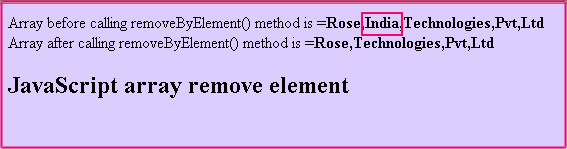Tutorials

# JavaScript array remove an element

As in the previous section of JavaScript array tutorials we have discussed.

As in the previous section of JavaScript array tutorials we have discussed.

# JavaScript array remove an element

As in the previous section of JavaScript array tutorials we have discussed that we can create a user defined function in JavaScript which will remove an array element by the provided index, now we will show you that how a user can remove an array element by providing the element's value.

In this example we have created a function removeByElement() which takes array object name and one array element's value which is to be removed and eliminates the specified array elements value. Here is the function definition as :

 function removeByElement(arrayName,arrayElement)  {   for(var i=0; i

Above lines of code defines the function for removing elements according to the value and for this purpose we have used splice() method. Here is the example code:

javascript_array_remove_element.html

    JavaScript array remove element example

JavaScript array remove element

After running this HTML code on the browser we will get the following output: